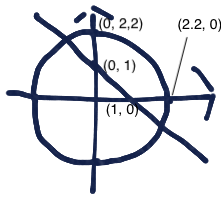# System of Nonlinear Equations

## Question

Find the value or values of that are solutions to this system of equations:

## Solution

If the equations look familiar enough so that you can sketch them by hand, then doing so may help you get your arms around the problem. (The calculator provided for the exam does not have graphing capability, so if you want to see a graph you pretty much need to make it yourself. Learn more about it here.)A very rough sketch will do. I think my sketch (left) is pretty crude. For this problem, the first equation is for a circle with center at the origin and radius equal to the square root of 5. The square root of 5 is about 2.2, so the circle goes through points (2.2, 0); (0, 2); (-2.2, 0); and (0, -2.2), approximately.

The second is for a line with a slope of -1 and intercepts at   and . The solutions to the system lie at the points of intersection of the graphs, one in the second quadrant (where is negative and is positive) and the other in the fourth quadrant (where is positive and is negative).

The sketch is just to help you get your arms around the problem. If it doesn’t work for you, you can skip it — it’s not essential.

You can solve using substitution. If  then ; substitute  for  in the equation

 Simplify: Multiply out, subtract 5 from each side, and collect like terms. Divide both sides by 2. Factor Set each factor equal to 0 and solve.

That’s all you need to answer the question.

To check your answers, use the equation  to find the y-values that correspond to the x-values you found, and then substitute x- and y-values into the equation

This question is about nonlinear systems of equations. (The whole system gets labeled nonlinear even though one of the equations is linear.)